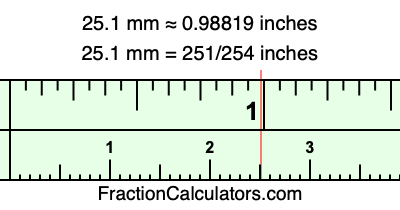25.1 mm in inchesHere is how to convert 25.1 mm to inches. We will give you the fractional answer, the decimal answer, and illustrate the answer on our tape measure.

One millimeter is equal to 5/127 inches, and 25.1 as a fraction is 251/10. Therefore, to get the fractional answer to 25.1 mm in inches, we multiply 251/10 by 5/127 and simplify if necessary.

cm × 5/127 = inches
251/10 × 5/127 = inches
251/10 × 5/127 = 251/254
251/10 mm = 251/254 inches
25.1 mm = 251/254 inches

As promised above, we also have the answer to 25.1 mm in inches in decimal form. Although the fractional answer above is exact, the decimal answer may be rounded if necessary:

251/254 ≈ 0.988188976377953
251/254 ≈ 0.98819
25.1 mm ≈ 0.98819 inches

Our image below shows 25.1 mm on a tape measure. The top row of the tape measure is inches, and each mark on the bottom row is 1 millimeter (10 millimeter marks equal 1 centimeter).As you can see, we drew a red line where 25.1 mm and 0.98819 inches meet on the measuring tape.

Millimeters to Inches Calculator
Here you can convert another length in millimeters to inches.

Convert  mm to inches.

What is 25.2 mm in inches?
Here is another inches to millimeters calculation that we have done for you.